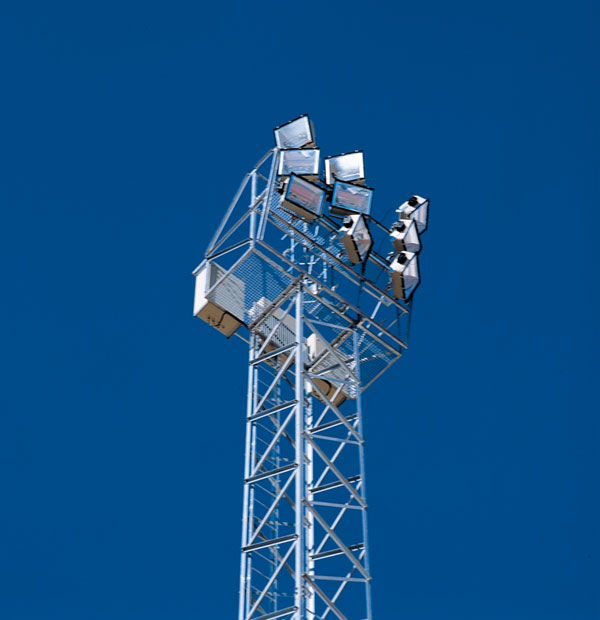STREET LIGHTING

These are “latticework” type masts  to install of platforms with interior ladder with rest platforms.

Hot dip galvanized according UNE EN ISO 1461.

AZ900-AZ1000 Models

AZ900

Height: From 12 to 30 meters.

Floodlights on platform: Equal or less than 9.

AZ1000

Height: From 12 to 42 meters.

Floodlights on platform: Equal or less than 10.

Upon request, they are supplied AZ-2000 and 3000, efforts 200-3000 daN.

Optional accesories:

• Approved anti-fall system or lifeline.
• Guardrail in the interior ladder.

Note: The masts are delivered unmounted, with assembly instructions enclosed.

Masts are made to support the wind loads calculated in the R.E.A.T.

Foundations

For guidance purposes only, the following table shows the details on the monoblock-type measurements that these metallic support structures require, according to the height (H) and the average coefficient of compressibility of the soil at a depth of two metres (K).

In spite of the adoption of one value or the other, the value must be calculated for each case and taking into account the real conditions of the soil and the expected tower stress.

The Sultzberger formula has been used to determine the values of the foundation measurements for each type of soil.FOUNDATIONS

These dimensions are given for informative purposes, the calculation and correct execution of the foundation being the responsibility of the project management.

TERRENO FLOJO K=8 Kg/cm3TERRENO FLOJO K=8 Kg/cm3TERRENO NORMAL K=12 Kg/cm3TERRENO NORMAL K=12 Kg/cm3TERRENO DURO K=16 Kg/cm3TERRENO DURO K=16 Kg/cm3
MODELOALTURAh (m)a (m)h (m)a (m)h (m)a (m)
AZ-90012 m2.101.00h=1.80 ma=1.00 mh=1.60 ma=1.00 m
AZ-90015 m2.101.10h=1.90 ma=1.05 mh=1.70 ma=1.05 m
AZ-90018 m2.251.10h=1.97 ma=1.05 mh=1.70 ma=1.05 m
AZ-90020 m2.251.20h=2.05 ma=1.10 mh=1.85 ma=1.10 m
AZ90022 m2.251.25h=2.05 ma=1.15 mh=2.00 ma=1.20 m
AZ90024 m2.301.30h=2.10 ma=1.15 mh=2.00 ma=1.10 m
AZ90026 m2.351.30h=2.15 ma=1.15 mh=2.00 ma=1.10 m
AZ90028 m2.351.35h=2.15 ma=1.25 mh=2.00 ma=1.15 m
AZ90030 m2.351.50h=2.15 ma=1.35 mh=2.05 ma=1.15 m
AZ100012 m2.201.15h=2.00 ma=1.10 mh=1.80 ma=1.10 m
AZ100015 m2.251.30h=2.10 ma=1.15 mh=1.95 ma=1.10 m
AZ100018 m2.301.45h=2.20 ma=1.20 mh=2.00 ma=1.15 m
AZ100020 m2.301.52h=2.20 ma=1.25 mh=1.05 ma=1.20 m
AZ100022 m2.301.62h=2.20 ma=1.35 mh=2.05 ma=1.30 m
AZ100024 m2.331.68h=2.25 ma=1.36 mh=2.09 ma=1.30 m
AZ100026 m2.381.70h=2.29 ma=1.40 mh=2.14 ma=1.31 m
AZ100028 m2.411.75h=2.32 ma=1.43 mh=2.15 ma=1.37 m
AZ100030 m2.451.77h=2.35 ma=1.47 mh=2.19 ma=1.39 m
AZ100032 m2.451.80h=2.35 ma=1.49 mh=2.20 ma=1.40 m
AZ100034 m2.451.84h=2.35 ma=1.53 mh=2.20 ma=1.40 m
AZ100036 m2.451.91h=2.35 ma=1.60 mh=2.20 ma=1.50 m
AZ100038 m2.451.98h=2.35 ma=1.66 mh=2.20 ma=1.56 m
AZ100040 m2.452.04h=2.35 ma=1.73 mh=2.20 ma=1.63 m
AZ100042 m2.452.11h=2.35 ma=1.79 mh=2.20 ma=1.69 m